# SSAT Middle Level Math : How to multiply fractions

## Example Questions

1 2 23 24 25 26 27 28 29 31 Next →

### Example Question #861 : Common Core Math: Grade 4

Select the answer choice that is equal to the following: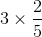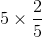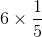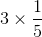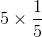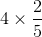Explanation:

When we multiply a whole number by a fraction, we multiply the whole number by the numerator, and the denominator stays the same.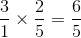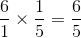### Example Question #1131 : Common Core Math: Grade 5

Solve the following: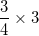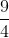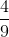Explanation:When we multiply a fraction by a whole number, we first want to make the whole number into a fraction. We do that by putting the whole number over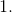Then we multiply like normal.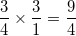### Example Question #811 : Fractions

Solve the following: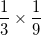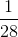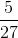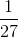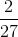Explanation:

When we multiply fractions, we multiply the numerator by the numerator and the denominator by the denominator.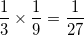### Example Question #1 : Solve Word Problems Involving Multiplication Of A Fraction By A Whole Number: Ccss.Math.Content.4.Nf.B.4c

Linda is having her friends over for a game night. If each person at the game night will drinkof a liter of soda, and there will bepeople at the game night, how many liters of soda will Linda need? Select the choice with the whole numbers that your answer will be between.andandandandandandExplanation: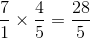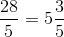becausecan go intofive times, withleft over.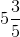is between the whole numbersand1 2 23 24 25 26 27 28 29 31 Next →

### All SSAT Middle Level Math Resources